# Backtesting Long Short Moving Average Crossover Strategy in Excel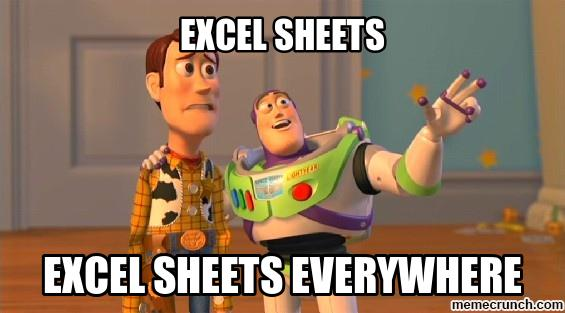By Jacques Joubert

Now for those of you who know me as a blogger might find this post a little unorthodox to my traditional style of writing, however in the spirit of evolution, inspired by a friend of mine Stuart Reid (TuringFinance.com), I will be following some of the tips suggested in the following blog post.

Being a student in the EPAT program I was excited to learn the methodology that others make use of when it comes to backtesting. As usual, we start off in by backtesting Excel and then migrate to R.

Having previously written a blog series on backtesting in Excel and then moving to R, I was very interested to see a slightly different method used by the QuantInsti team.

“By calculating transaction prices it opens up some very interesting doors for implementing MAE analysis”

The one main difference in the method is that it opens the door for performance metrics like:

• Total Positive returns
• Negative Returns
• Hit Ratio
• Average return

But suffers from not being able to plot an equity curve like my original method (that I like to think of us a vectorised backtest), you can however, easily incorporate the equity curve, as I did in this post.

Build the “Hello World” of trading strategies: the “Long Short Moving Average Crossover Strategy”.#### Step 1: Get data

There are several places from which you can get data, however for this example we will get data from Yahoo Finance. I will be building this example using Google as a share.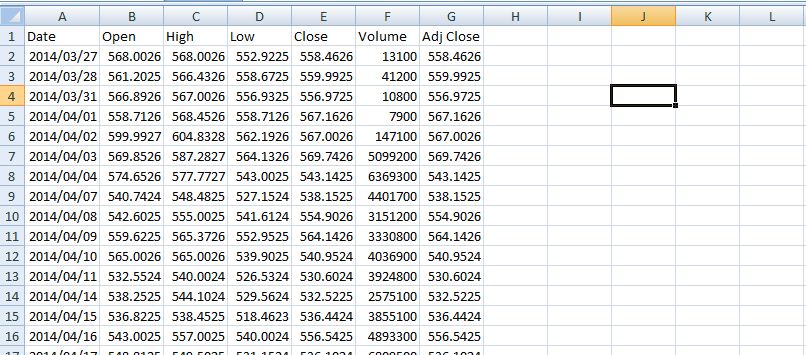Price data from Yahoo in CSV file format

#### Step 2: Create a column for both the long and the short simple moving average (SMA)

For this example I want you to make use of the 5 and 25 day SMA. For those of you who are new to trading strategies, a SMA is simply the total sum of closing price divided by the number of observations.

2.1) Create the short term SMA (5 days)

Using the following formula in Excel: =AVERAGE(G2:G6)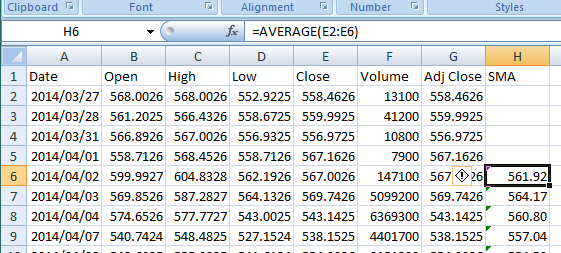2.2) Create the long term SMA (25 Days)

Using the following formula in Excel: =AVERAGE(E2:E26)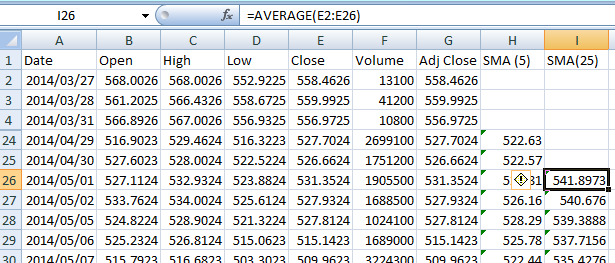#### Step 3: Generate trading signals

It is at this step where readers will pick up on a major difference from my previous blog posts on building a vectorised backtester. I will incorporate my original methodology in this post as well in order to plot the equity curve.

The next thing we need to do is to generate buy and sell signals

Logic for Step 3:

If:

In the previous day the (5)SMA was below the (25)SMA and in the current day there is a change where the (5)SMA is now above the (25)SMA,

Then:

Write the string “BUY” in the current field

Else If:

In the previous day the (5)SMA was above the (25)SMA and in the current day there is a change where the (5)SMA is now below the (25)SMA,

Then:

Write the string “SELL” in the current field

Else:

Add an empty string “” to the current field.

This is represented in Excel using the following formula: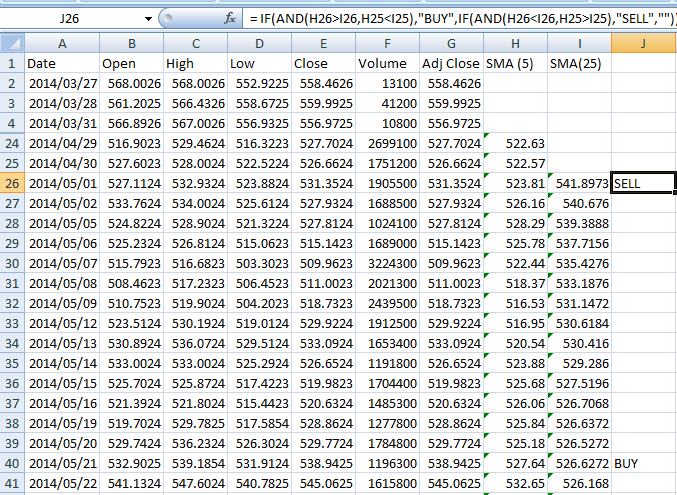Note:

The SMAs are calculated on closing prices and not adjusted close because we want the trade signal to be generated on the price data and not influenced by dividends paid.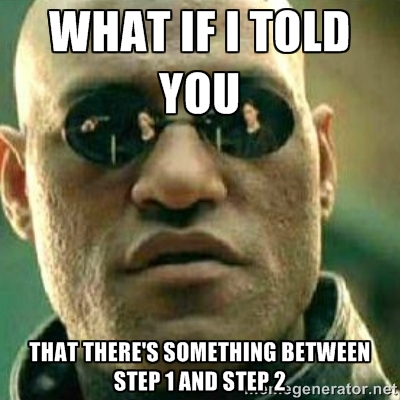#### Step 4: Get purchase / selling price of the trade

In the next column add the following Excel formula: =IF(J26<>"",G27,K26)

The logic is as follows:

If the trade signal column for the previous day (Very important to lag the indicator as to remove look-ahead bias) is not an empty string then make use of the previous price above the current field, else set the current field to the closing price for the day.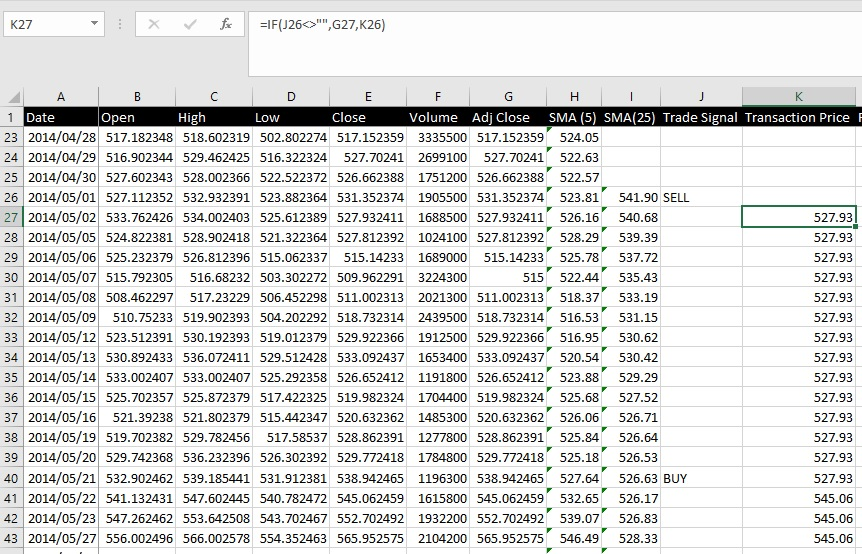Note:

Some may argue that you can’t actually get the close for the day but you can if you put your order in at the closing auction, and even after the auction there are some residual orders that you can fill, one of the previous funds I worked for did exactly this.

#### Step 5: Calculate returns

Add a column called returns that makes use of the following Excel Formula: =IF(J26="SELL",K27/K26-1,IF(J26="BUY",1-K27/K26,""))

Logic:

If the previous day generated a SELL signal then take today’s closing price and divide it by the purchase price and subtract 1.

Else If the previous day generated a BUY signal then add 1 and subtract (today’s closing price and divide it by the purchase price).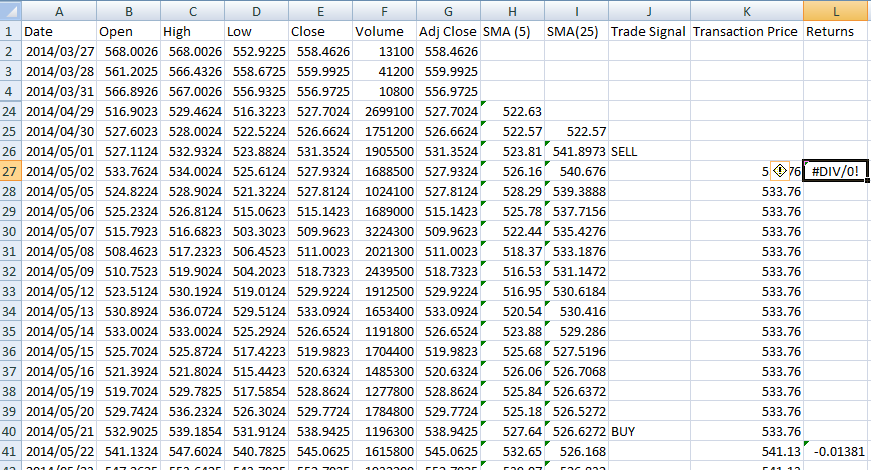This formula calculates the returns for a given trade.

#### Step 6: Calculate some performance metrics

• Positive returns: =SUMIF(L:L,">0")
• Negative returns: =SUMIF(L:L,"<0")
• Hit Ratio =O4/(O4+O5)
• Average Returns =AVERAGE(L:L)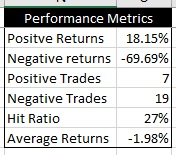These aren’t the traditional portfolio performance metrics but by calculating the purchase and selling price it opens up some very interesting doors for implementing maximum adverse excursion analysis that can be used to optimise stop losses.

“Note: I wasn’t able to calculate these metrics in my previous method due to not having recorded the purchase and sale prices of transactions.”#### Step 1: Add two new columns for the Daily returns and the natural log daily returns of the share

For this I will make use of the adjusted closing price as I want dividends paid to be reflected in our strategies equity curve and total return profile.

Formula for Daily Returns is: (Today’s Price / Yesterday’s Price) - 1

Excel formula: =G3/G2-1

The formula is use for the natural log daily returns is: LN(Today’s price / Yesterday’s price)

Excel formula: = LN(G3/G2)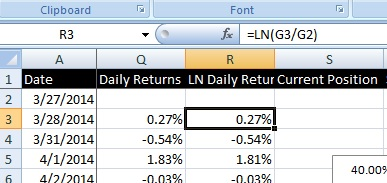#### Step 2: Calculate the Long or Short holdings signals

In this column we want to know if we are currently holding a long or a short position. This is represented by 1 for long and -1 for short.

This builds on the moving average cross over strategy by going long if the short term SMA is above the long term SMA and short if the opposite is true.

“Note: you have to lag the signals by one day in order to remove look-ahead bias.”

In this example the Excel formula is as such: =IF(H26>I26, 1, -1)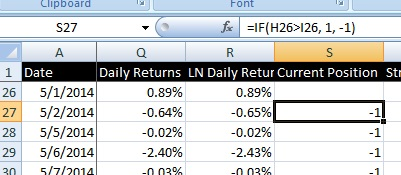#### Step 3: Calculate Strategy ln Daily Returns

This is the easy part, simply multiply the natural log daily return by the current position.

Excel formula: =R27*S27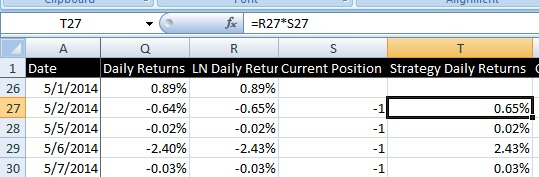#### Step 4: Calculate the cumulative returns for both the strategy and the share as if you bought and hold. (Do this to act as a comparison)

The formula to cumulate returns is simple, for LN returns you simply add them using =T27+U26.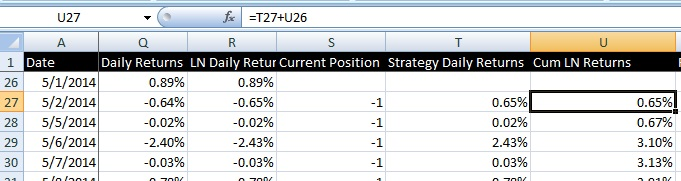Next you need to reverse the natural log using the following formula: =EXP(U27)-1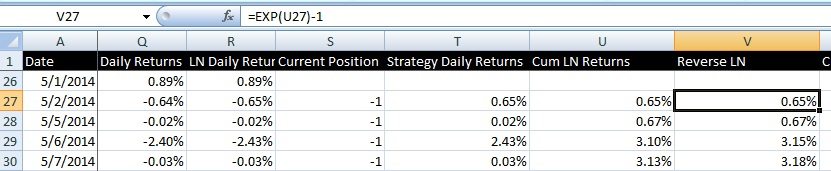And then you need to calculate the stocks cumulative returns:

Excel formula =(1+Q27)*(1+Q26)-1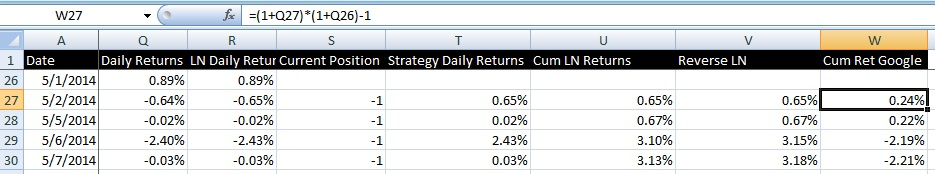#### Step 5: Plot the returns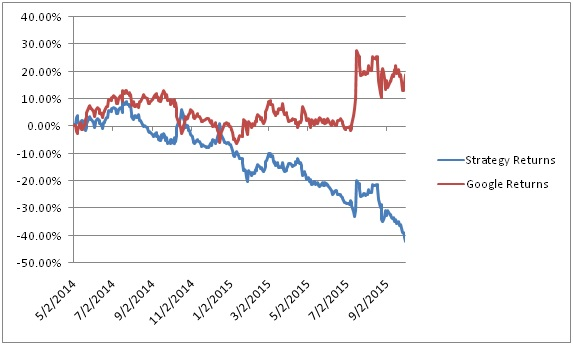As can be seen from the chart above, this strategy isn’t a profitable one on this particular time frame and share but this . I would encourage readers to explore other trading strategies by trying to incorporate the RSI indicator to act as a guide on how to size a position.### Next Steps

After backtesting in Excel, learn to import and backtest on Zipline using data from Google and OHLC data in CSV format. Calculate backtesting results such as PnL, number of trades, etc. Click here to start now.

Update

We have noticed that some users are facing challenges while downloading the market data from Yahoo and Google Finance platforms. In case you are looking for an alternative source for market data, you can use Quandl for the same.